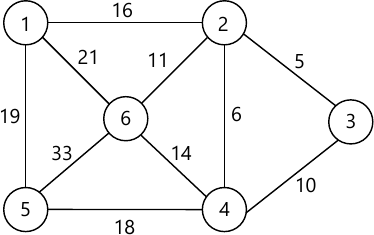MORE IN Data Structures & Algorithms
SPPU Electronics and Telecom Engineering (Semester 3)
Data Structures & Algorithms
December 2015
Total marks: --
Total time: --
INSTRUCTIONS
(1) Assume appropriate data and state your reasons
(2) Marks are given to the right of every question
(3) Draw neat diagrams wherever necessary

Solve any one question from Q1 and Q2
1 (a) Write a C function for linear search. Discuss its time complexity.
6 M
1 (b) How can a polynomial be stored using an array? Explain with example.
6 M

2 (a) Explain parameter passing by value and passing parameter by reference with suitable example.
6 M
2 (b) Explain selection sort algorithm.
6 M

Solve any one question from Q3 and Q4
3 (a) What is doubly linked list? Explain insert operation in doubly linked list.
6 M
3 (b) Evaluate the following postfix expression using stack
6 2 3 + - 3 8 2 / + * 2 ∧
(note: ∧ stands for power and all operands are single digit).
7 M

4 (a) What is singly linked list? Explain traversal operation in singly linked list.
7 M
4 (b) Write a short note on circular queue. Compare it with linear queue.
6 M

Solve any one question from Q5 and Q6
5 (a) What is Binary Search Tree (BST)? Explain the following operations in BST:
i) Searching a value is BST
ii) Inserting a new value in BST.
7 M
5 (b) What is AVL tree? Define Balance factor. Explain RR rotation with an example.
5 M

6 (a) What is Binary Search Tree (BST)? Construct a BST for the following numbers:
47, 55, 23, 17, 39, 11, 50, 9, 19, 74, 33, 28
Show all the steps.
Write its preorder traversal.
8 M
6 (b) Explain threaded binary tree with an example. What is its advantage?
4 M

Solve any one question from Q7 and Q8
7 (a) Write a C function to implement 'Breadth First Search' traversal of a graph implemented using adjacency matrix.
6 M
7 (b) What do you mean by indegree and outdegree of a vertex in a graph? Write a C function to find indegree and outdegree of a vertex in a graph implemented using adjacency matrix.
7 M

8 (a) Define the term Graph. With the help of suitable example give adjacency matrix representation and adjacency list representation of the graph.
7 M
8 (b) What do you mean by spanning tree of a graph? Find the minimal spanning tree of the following graph using Prim's algorithm.6 M

More question papers from Data Structures & Algorithms